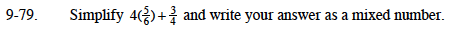### Home > MC1 > Chapter 9 > Lesson 9.2.3 > Problem9-79

9-79.$\frac{4}{1}\left(\frac{5}{6}\right)+\frac{3}{4}$

$\frac{20}{6}+\frac{3}{4}$

Use Giant Ones to get

$\frac{?}{12}+\frac{?}{12}$

$\frac{40}{12}+\frac{9}{12}$

$\frac{49}{12}=4\frac{1}{12}$